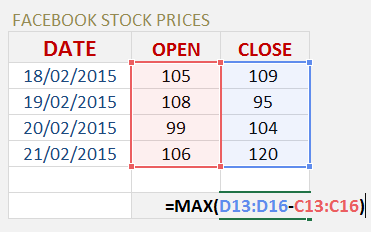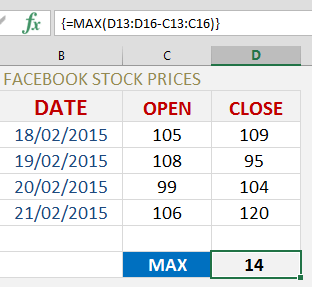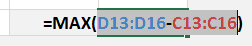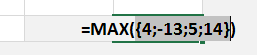An Array Formula performs an Excel operation (math, comparative, join or function argument) on an array or range of data.  This could be a range of cells, a worksheet reference or a defined name.

An Array contains more than one cell, so you cannot perform an Array Formula on a single cell.

To turn your formula into an Array Formula, you will need to press CTRL+SHIFT+ENTER which will put the squiggly brackets {} outside the formula:

{=MAX(D13:D16C13:C16)}

Let’s break down the different Excel operations that you can use to create an Array Formula:

MATH: +-*/()

COMPARATIVE: =<>

JOIN: &

FUNCTIONS: MAX, MIN, IF, INDEX, VLOOKUP etc

Let’s do an example of an Array Formula that calculates the maximum stock value change over a four day period:

STEP 1: Enter the MAX formula =MAX(

STEP 2: Subtract one array/range of data from another array/range of data =MAX(D13:D16C13:C16)STEP 3: Instead of pressing ENTER to evaluate the formula, you need to press CTRL+SHIFT+ENTER to turn the formula into an Array Formula which will look like this: {=MAX(D13:D16C13:C16)}STEP 4: By pressing F9 on the selected formula array will give you the resulting array of numbers (press CTRL+Z to get out of this mode when you are done checking the formula results):If we had to get the above result using a non-Array Formula we would have to create a helper column which subtracts the Open & Close cells and then enter the MAX formula to reference these results.  This is double the work!

Have a look at the following tutorial on the two methods: